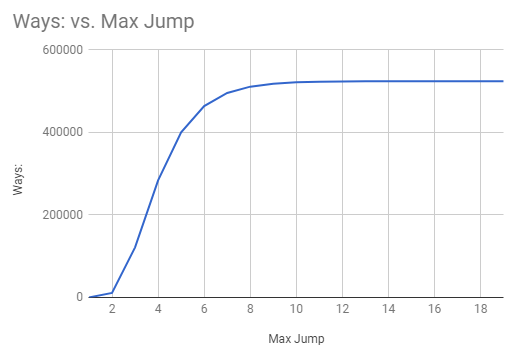A frog needs to jump across 20 lily pads. He starts on the shore (Number 0) and ends precisely on the last lily pad (Number 20). He can jump one or two lily pads at a time. How many different ways can he reach his destination?

What if he can jump one, two or three at a time? Or four? Five? Six? Etc.

(fivethirtyeight)

## Solution

### 1. The frog can jump one or two pads at a time.

There is just one way of traversing zero lily pads (stay on the shore), and just one of traversing a single pad: jump to it.

For any other number $n$ of pads, the number of ways of traversing $n$ pads is simply the sum of the numbers of ways of traversing $n-1$ and $n-2$ pads. That’s because every way of traversing $n$ pads either starts with a jump to the first pad and is completed by a way of traversing $n-1$ more pads, or starts with a jump to the second pad and is completed by a way of traversing $n-2$ more.

So the sequence, for $n$ from $0$ to $20$, of the numbers of ways of traversing $n$ pads, is the start (or the start after the first element, if the first element is $0$) of the Fibonacci Sequence: $1,1,2,3,5,8, \ldots, 10946$.

We can calculate that in $19$ simple two-integer sums, or we can use the following closed-form expression (if you find exponentiation to be an improvement over the very simple recurrence, which at least in this case is questionable):

$\frac{1}{\sqrt{5}}\left[\left(\frac{1+\sqrt{5}}{2}\right)^{21} - \left(\frac{1-\sqrt{5}}{2}\right)^{21}\right]$

### 2. The frog can jump from one to m pads at a time.

Let $W_m(n)$ be the number of ways of traversing $n$ lily pads, given that the frog can jump up to $m$ at a time.

For any $m$, $W_m(0) = 1$ and for $n>0$, $W_m(n)$ is the sum of $W_m(i)$, where $i$ ranges over the up-to-$m$ positive integers that precede $n$:

$W_m(n) = \sum_{i=\max(0,n-m)}^{n-1} W_m(i)$

This yields a generalization of the Fibonacci Sequence, known as the $n$-Step Fibonacci Sequence. The values of $W_m(20)$ are listed below. As a sanity-check, we can verify that $W_{20}(20)$ is $2^{19}$, as it should be, because that’s how many possible choices of stopping-pads there are on the way to the last lily pad.### Code (Python)

N = 20
W = {}
for m in range(1,N+1):
W[(0,m)] = 1
for n in range(1,N+1):
for m in range (1,N+1):
W[(n,m)] = 0
for i in range(max(0,n-m),n):
W[(n,m)] += W[(i,m)]
print "Max Jump, Ways:"
for m in range(1,N+1):
print m,",", W[(N,m)]


Output:

Max Jump, Ways:
1 , 1
2 , 10946
3 , 121415
4 , 283953
5 , 400096
6 , 463968
7 , 495776
8 , 510994
9 , 518145
10 , 521472
11 , 523008
12 , 523712
13 , 524032
14 , 524176
15 , 524240
16 , 524268
17 , 524280
18 , 524285
19 , 524287
20 , 524288
[Finished in 0.1s]